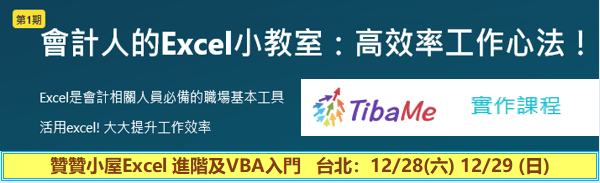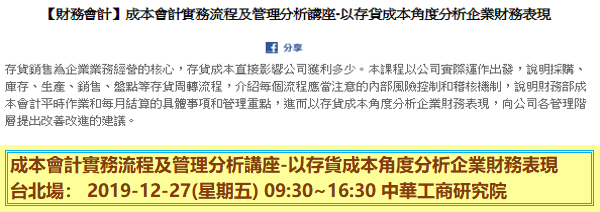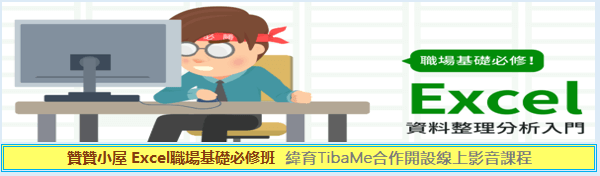VBA如何依照所選擇年份幣別設定網址Sub IfFunction()

Dim i As Integer, Month, WebAddress, DesCell As String

For i = 1 To 12

If i < 10 Then

Month = “0” & CStr(i)

Else

Month = CStr(i)

End If

WebAddress = “URL;http://rate.bot.com.tw/xrt/quote/2015-” & Month & “/USD”

DesCell = Cells((1 + (i – 1) * 25), 1).Address

Cells(i, 1).Value = Month

Cells(i, 2).Value = DesCell

Cells(i, 3).Value = WebAddress

Next i

End Sub